# Queue in C Programming

###### Data Structure in C

In this lesson, we will understand what is Queue in C Programming and how to create them along with some examples.

## What is Queue in C

A Queue in C is a data structure in which we can add element only at one end, called the rear of the queue, and delete element only at the other end, called the front of the queue.

We can see the example of a queue in our daily life as the queue of people.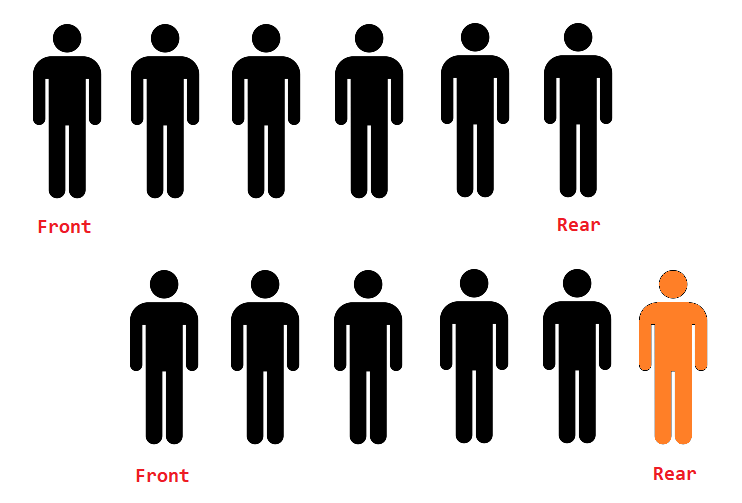In the above image, we can see that the new people can join the queue from the rear end and, when the work is over, the people at the front will leave first.## Operation on Queue

There are two operations possible on the queue.

• Delete - When we delete an element from the queue.

To understand how the above operations work on a queue. See the example given below.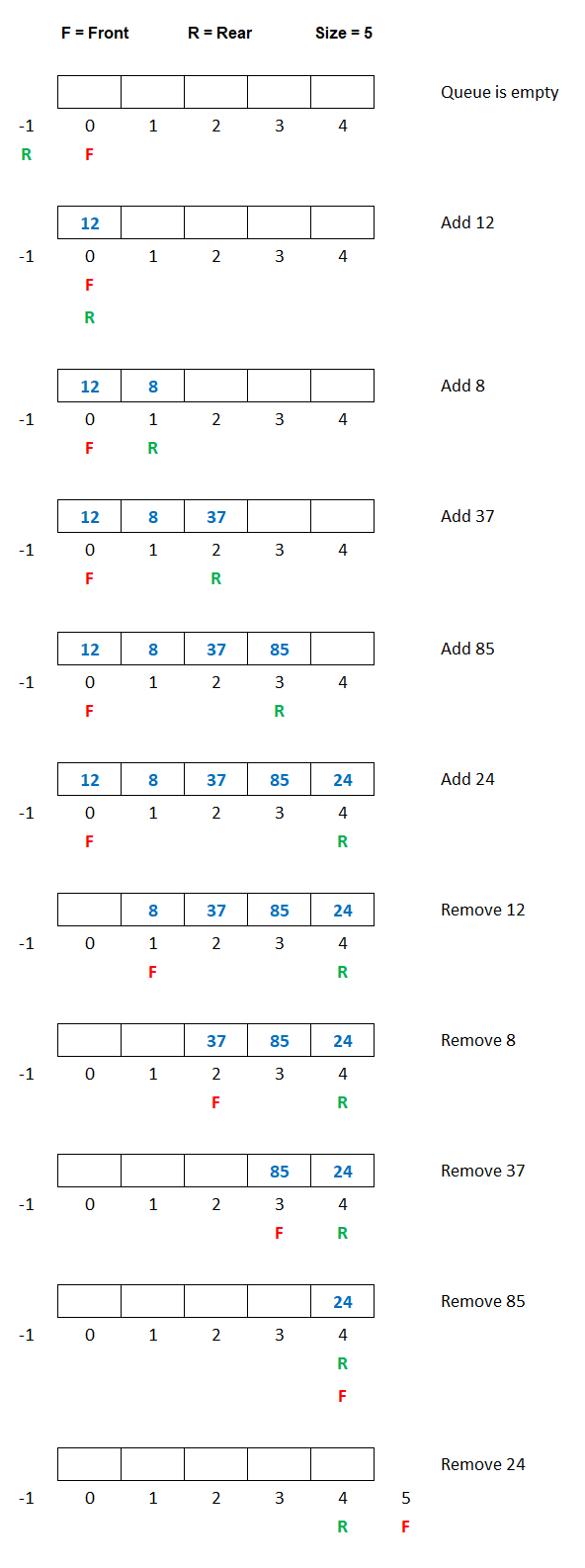From the above image, we can see that when we add a new element in the queue, the variable R is increased by 1, and the new element is added at the new position of R. Similarly, when we delete an element from the queue, the variable F is increased by 1.

The queue behaves like a first in first out manner. It means that the elements that are added first to the queue, are removed first from the queue.

So a queue is also known as FIFO (First In First Out) data structure.

## Implementation of Queue in C

The queue in C programming can be implemented in two ways using:

• Array

In this lesson, we will see the implementation of a queue using an array. We will also discuss queue using a single linked list later on in the subsequent lesson.

## Array Implementation of Queue

Since a queue is a collection of the same type of elements, so we can implement the queue using an array.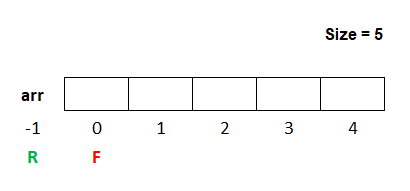In the above image, we can see an array named arr whose size is 5. We take two variables R and F, The variable R stands for rear and the default value is -1. The variable F stands for front and the default value is 0.

For add operation in the queue first, we check if the value of R is equal to the value of size-1 then, we will display a message Queue is full, else we will increase the value of R by 1 and add the element in the array at the new location of R.

#### Example

``````if(R==size-1)
{
printf("Queue is full\n");
}
else
{
R=R+1;
arr[R]=new_item;
}``````

If we add three elements, say 12, 15 and 26 in the queue, then the queue will look like as shown in the image below.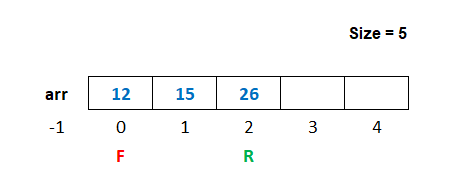### Delete Operation in Queue

For delete operation in the queue first, we check if the value of F is greater than the value of R then, we will display a message Queue is empty, else we will display the deleted element on the screen and then increase the value of F by 1.

#### Example

``````if(F>R)
{
printf("Queue is empty\n");
}
else
{
printf("Element Deleted = %d",arr[F]);
F=F+1;
}``````

If we delete the first elements 12 from the queue, then the queue will look like as shown in the image below.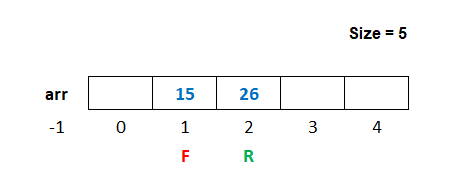## Program of Queue using Array

Below is the complete program of queue in C using an array having size 5.

#### Queue Program in C using Array

``````#include <stdio.h>
#include <conio.h>
#include <stdlib.h>
#define size 5

int main()
{
int arr[size],R=-1,F=0,ch,n,i;

for(;;)		// An infinite loop
{
system("cls");		// for clearing the screen
printf("2. Delete\n");
printf("3. Display\n");
printf("4. Exit\n");
printf("Enter Choice: ");
scanf("%d",&ch);

switch(ch)
{
case 1:
if(R==size-1)
{
printf("Queue is full");
getch();	// pause the loop to see the message
}
else
{
printf("Enter a number ");
scanf("%d",&n);
R++;
arr[R]=n;
}
break;

case 2:
if(F>R)
{
printf("Queue is empty");
getch();	// pause the loop to see the message
}
else
{
printf("Number Deleted = %d",arr[F]);
F++;
getch();	// pause the loop to see the number
}
break;

case 3:
if(F>R)
{
printf("Queue is empty");
getch();	// pause the loop to see the message
}
else
{
for(i=F; i<=R; i++)
{
printf("%d ",arr[i]);
}
getch();	// pause the loop to see the numbers
}
break;

case 4:
exit(0);
break;

default:
printf("Wrong Choice");
getch();	// pause the loop to see the message
}
}
return 0;
}``````

In the above program, we have defined a macro named size having value 5 using the statement #define. We can use the word size to declare the size of the array as int arr[size]. When we run the above program, the word size will be replaced by its value 5. So the size of the array will be 5.